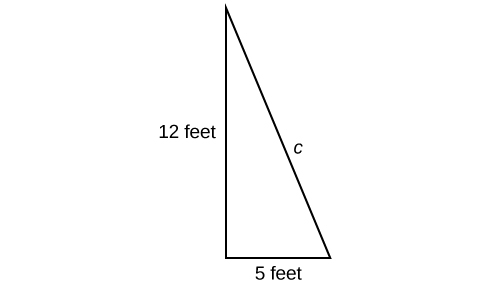## Introduction to Radicals and Rational Expressions

### Learning Objectives

By the end of this section, you will be able to:

• Evaluate square roots.
• Use the product rule to simplify square roots.
• Use the quotient rule to simplify square roots.
• Add and subtract square roots.
• Rationalize denominators.
• Use rational roots.

A hardware store sells 16-ft ladders and 24-ft ladders. A window is located 12 feet above the ground. A ladder needs to be purchased that will reach the window from a point on the ground 5 feet from the building. To find out the length of ladder needed, we can draw a right triangle as shown in Figure 1, and use the Pythagorean Theorem.Figure 1

$\begin{array}{ccc}\hfill {a}^{2}+{b}^{2}& =& {c}^{2}\hfill \\ \hfill {5}^{2}+{12}^{2}& =& {c}^{2}\hfill \\ \hfill 169& =& {c}^{2}\hfill \end{array}$

Now, we need to find out the length that, when squared, is 169, to determine which ladder to choose. In other words, we need to find a square root. In this section, we will investigate methods of finding solutions to problems such as this one.